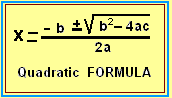Posted on: April 15, 2020 Posted by: Susan Johnsey Comments: 0With the variable as x and the a, b, and c the numbers in the quadratic equation

ax^2 +bx +c = 0

we can find the value for x that will make the above equation true by using this formula:

x = \frac{-b\pm\sqrt{b^2 -4ac} }{2a}

Solve the equation below. Do you see the right side is 0? One side must be 0. Then we must decide a, b, and c. { a (multiplied by x^2) is 3 and the b (multiplied by x) is -1 and c (the “lone number” is -2}